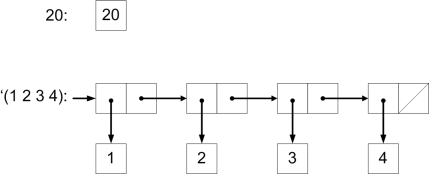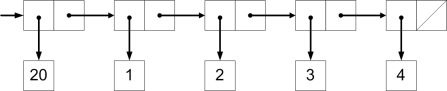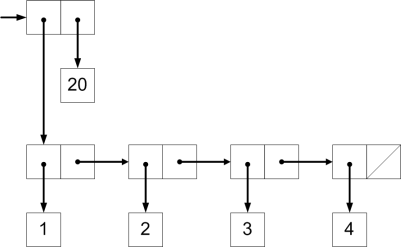Tags SICP

The authors start this section by presenting the “Closure Property” of Lisp’s data combinator `cons`. Note that this isn’t what’s usually implied by the term “closure” in describing programming languages. This is explained in the book:

The ability to create pairs whose elements are pairs is the essence of list structure’s importance as a representational tool. We refer to this ability as the closure property of `cons`. In general, an operation for combining data objects satisfies the closure property if the results of combining things with that operation can themselves be combined using the same operation.

And there is a clarification in footnote 6:

The use of the word “closure” here comes from abstract algebra, where a set of elements is said to be closed under an operation if applying the operation to elements in the set produces an element that is again an element of the set. The Lisp community also (unfortunately) uses the word “closure” to describe a totally unrelated concept: A closure is an implementation technique for representing procedures with free variables. We do not use the word “closure” in this second sense in this book.

It is very curious that the more familiar usage of the word “closure” (which usually means a function with the values of free variables captured from the enclosing lexical context) is discouraged by the authors of SICP. I can only guess that the traditional meaning is indeed the one defined in the book, since it fits the mathematical meaning of “closure” better. I will try to use the full term “closure property” to avoid confusion, whenever possible.

#### Exercise 2.17

```(defun last-pair (items)
(if (null (cdr items))
items
(last-pair (cdr items))))
```

#### Exercise 2.18

```(defun my-reverse (items)
(if (null items)
nil
(append
(my-reverse (cdr items))
(list (car items)))))
```

#### Exercise 2.19

This exercise is a definite antithesis to anyone who claims that the exercises in SICP are too difficult :-) The authors basically chop and chew the answer and feed it to you with a spoon. It would have been more challenging to try and solve it without having the skeleton of `cc` in front of the eyes.

```(defun no-more? (coins)
(null coins))

(defun except-first-denomination (coins)
(cdr coins))

(defun first-denomination (coins)
(car coins))

(defun cc (amount coin-values)
(cond ((= amount 0) 1)
((or (< amount 0) (no-more? coin-values)) 0)
(t
(+  (cc amount
(except-first-denomination coin-values))
(cc (-  amount
(first-denomination coin-values))
coin-values)))))
```

The order of the list `coin-values` does not affect the answer of `cc`. To understand why this is so, note that the first recursive call will try to match the amount with all the possibilities, no matter what is their order.

#### Exercise 2.20

In Common Lisp the dotted-tail notation for specifying functions with arbitrary number of arguments is not supported. Instead, the specifier &rest is used to achieve the same purpose.

```(defun same-parity (elem &rest others)
(let ((result (list elem)))
(dolist (other others)
(when (= (rem elem 2) (rem other 2))
(setf result (append result (list other)))))
result))
```

This could’ve been implemented in dozens of ways – I chose to practice `dolist` because it’s so suitable for iteration over a list. I’m sure this can be done much more succinctly using `loop`.

Trivia: The test `(= (rem elem 2) (rem other 2))` can be rewritten as `(= 0 (rem (+ elem other) 2))` because the parity of the sum of two numbers is even IFF their parities are the same.

#### Exercise 2.21

Common Lisp doesn’t suffer from a shortage of mapping methods, that’s for sure ! `map, map-into, mapc, mapcan, mapcar, mapcon, maphash, mapl, maplist` – pick your favorite :-) To really understand the differences it is essential to read the documentation (the HyperSpec is particularly helpul, as usual). In my code I will just use the mapping method I find most suitable.

```(defun square-list-solo (items)
(if (null items)
nil
(cons (square (car items))
(square-list-solo (cdr items)))))

(defun square-list-map (items)
(mapcar #'square items))
```

In this case it’s `mapcar`, which is probably the function closest to Scheme’s `map` that’s used in the book.

#### Exercise 2.22

Here’s Luis’s function with an embedded printout to help understanding what’s going on:

```(defun square-list-iter (items)
(labels (
(format t "~A - ~A~%" things answer)
(if (null things)
(iter (cdr things)
(cons (square (car things))
(iter items nil)))

(print (square-list-iter '(1 2 3 4)))

=>

(1 2 3 4) - NIL
(2 3 4) - (1)
(3 4) - (4 1)
(4) - (9 4 1)
NIL - (16 9 4 1)

(16 9 4 1)
```

Since each new square value is consed in front of the existing answer, the result comes out reversed. It is as if you’re taking a deck of cards and start taking cards from the top and placing them in another deck, in order. What you’ll get in the end is a reversed deck.

When Luis reverses the order of arguments to `cons`:

```(defun square-list-iter (items)
(labels (
(format t "~A - ~A~%" things answer)
(if (null things)
(iter (cdr things)
(square (car things)))))))
(iter items nil)))

(print (square-list-iter '(1 2 3 4)))

=>

(1 2 3 4) - NIL
(2 3 4) - (NIL . 1)
(3 4) - ((NIL . 1) . 4)
(4) - (((NIL . 1) . 4) . 9)
NIL - ((((NIL . 1) . 4) . 9) . 16)

((((NIL . 1) . 4) . 9) . 16)
```

In some sense this is better because the order of the elements is right. However, cons builds a conventional list only when the new element is prepended to the existing list, not appended to its end. Consider this:

```> (cons 20 '(1 2 3 4))
(20 1 2 3 4)
> (cons '(1 2 3 4) 20)
((1 2 3 4) . 20)
```

If you think in terms of the box and pointer notation, what we have prior to consing is:What `(cons 20 '(1 2 3 4))` does is:While `(cons '(1 2 3 4) 20)` does this:#### Exercise 2.23

Interestingly, such a function exists in CL – it’s `mapc`, which doesn’t accumulate the result but just returns its argument:

```> (mapc #'print '(1 2 3 4))

1
2
3
4
(1 2 3 4)
```

`for-each` can also be simply defined in terms of `dolist`:

```(defun for-each (func items)
(dolist (item items)
(funcall func item)))

(for-each (lambda (x) (print x))
(list 57 321 88))
=>

57
321
88
```